# Edexcel A Level Chemistry:复习笔记1.6.1 Empirical & Molecular Formulae

### Empirical & Molecular Formula Definitions

• The molecular formula is the formula that shows the number and type of each atom in a molecule
• E.g. the molecular formula of ethanoic acid is C2H4O2

• The empirical formula is the simplest whole number ratio of atoms of each element present in one molecule or formula unit of a compound
• E.g. the empirical formula of ethanoic acid is CH2O

### Empirical & Molecular Formula Calculations

#### Empirical formula

• Empirical formula is the simplest whole number ratio of the elements present in one molecule or formula unit of the compound
• It is calculated from knowledge of the ratio of masses of each element in the compound
• The empirical formula can be found by determining the mass of each element present in a sample of the compound
• It can also be deduced from data that gives the percentage compositions by mass of the elements in a compound

#### Worked Example

Empirical formula from mass
Determine the empirical formula of a compound that contains 10 g of hydrogen and 80 g of oxygen.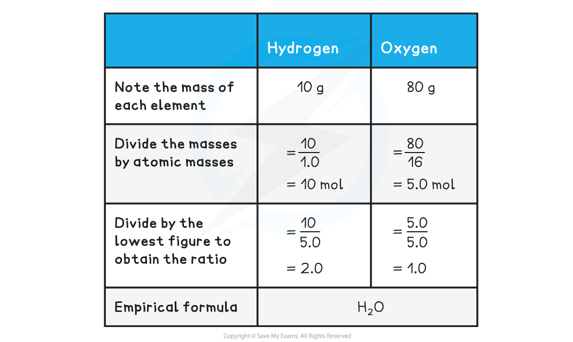• The above example shows how to calculate empirical formula from the mass of each element present in the compound
• The example below shows how to calculate the empirical formula from percentage composition

#### Worked Example

Empirical formula from %
Determine the empirical formula of a compound that contains 85.7% carbon and 14.3% hydrogen.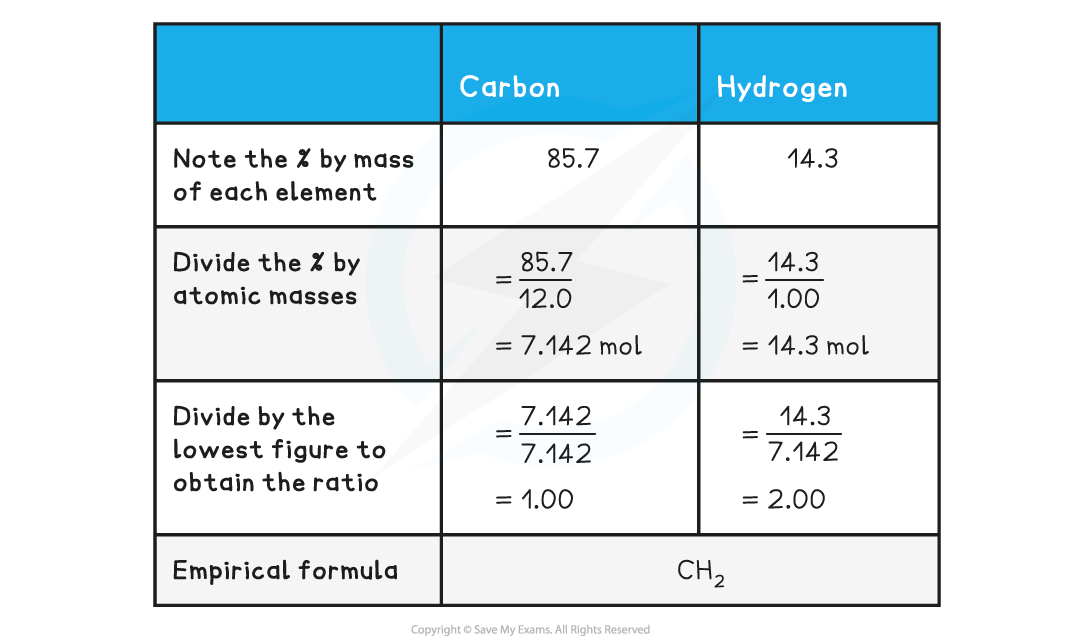#### Molecular formula

• The molecular formula gives the exact numbers of atoms of each element present in the formula of the compound
• The molecular formula can be found by dividing the relative formula mass of the molecular formula by the relative formula mass of the empirical formula
• Multiply the number of each element present in the empirical formula by this number to find the molecular formula

#### Worked Example

Calculating molecular formula
The empirical formula of X is C4H10S and the relative molecular mass of X is 180.2

What is the molecular formula of X?

(Ar data: C = 12.0, H = 1.0, S = 32.1 )

Step 1: Calculate relative mass of the empirical formula

• Relative empirical mass = (C x 4) + (H x 10) + (S x 1)
• Relative empirical mass = (12.0 x 4) + (1.0 x 10) + (32.1 x 1)
• Relative empirical mass = 90.1

Step 2: Divide relative formula mass of X by relative empirical mass

• Ratio between Mr of X and the Mr of the empirical formula = 180.2 / 90.1
• Ratio between Mr of X and the Mr of the empirical formula = 2

Step 3: Multiply each number of elements by 2

• (C4 x 2) + (H10 x 2) + (S x 2)     =    (C8) + (H20) + (S2)
• Molecular Formula of X is C8H20S2

#### Ideal Gas Equation

• The ideal gas equation is:

PV = nRT

• P = pressure in pascals (Pa)
• V = volume in cubic metres (m3)
• n = the amount of substance in moles (mol)
• R = the gas constant, which is given in the Data Booklet as 8.31 J mol-1 K-1
• T = temperature in Kelvin (K)

#### Exam Tip

There are several calculations in Chemistry where you need to convert the units to or from SI units

The ideal gas equation has three:

1. Pressure is often quoted in kPa but the calculation needs pressure in Pa
• kPa to Pa = multiply by 1000 or 103
2. Volume is usually quoted in cm3 or dm3 but the calculation needs volume in m3
• cm3 to m3 = divide by 1000000 or multiply by 10-6
• dm3 to m3 = divide by 1000 or multiply by 10-3
3. Temperature can be quoted in Kelvin or Celsius
• Celsius to Kelvin = + 273

This is why you should always show your working! Examiners can't take all of your marks for one error, if you show your working then they should check through for errors and award marks accordingly

• The ideal gas equation can be used to find the amount of moles in a gaseous substance
• It can also be used for volatile liquids at temperatures above their boiling point
• If the mass of the substance is known, then the molar mass can be calculated
• This can then be used with empirical formula data to determine the molecular formula of a compound

#### Worked Example

An unknown compound was analysed and found to contain 66.7% of carbon, 11.1% hydrogen and the remainder was oxygen

0.135 g of the unknown compound had a volume of 56.0 cm3 at a temperature of 90 oC and a pressure of 101 kPa

Determine the molecular formula of the unknown compound

Step 1: Calculate the number of moles of carbon, hydrogen and oxygen

 Carbon: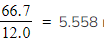moles Hydrogen: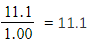moles Oxygen: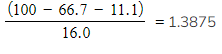moles

Step 2: Divide by the smallest answer to get the ratio

 Carbon: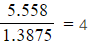Hydrogen: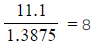Oxygen: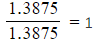Step 3: State the empirical formula

• The empirical formula is C4H8O

Step 4: Calculate the amount in moles, using PV = nRT

• n =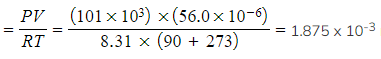moles

Step 5: Calculate the molar mass

• Molar mass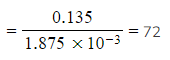Step 6: Deduce the molecular formula

• The empirical formula, C4H8O, has a mass of (4 x 12.0) + (8 x 1.0) + 16.0 = 72.0
• Therefore, the molecular formula is also C4H8O